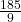## Change 20 5/9 to an improper fraction.

Question

Change 20 5/9 to an
improper fraction.

in progress 0
2 months 2021-07-25T07:02:52+00:00 2 Answers 2 views 0Step-by-step explanation:

1) The formula of changing mixed fractions to improper fractions is denominator times the whole number plus the numerator

2) Denominator = 9

Whole number = 20

Numerator = 5

9 x 20 + 5 = 185

Therefore, the answer isHope this helps!